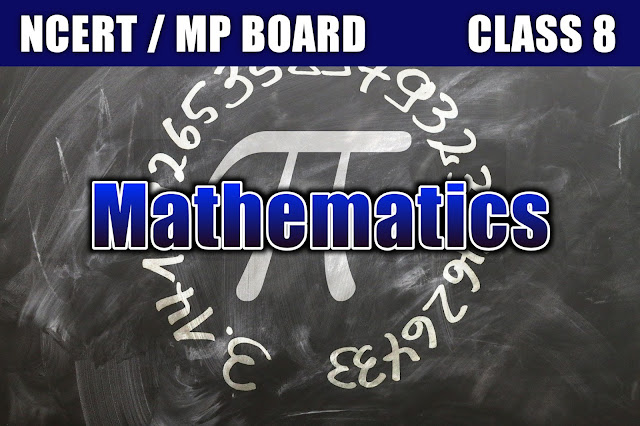# Class 8 Maths# CLASS 8# MathematicsChapter 1. Rational NumbersChapter 2. Linear Equations in One VariableChapter 3. Understanding Quadrilaterals Chapter 4. Practical GeometryChapter 5. Data HandlingChapter 6. Squares and Square RootsChapter 7. Cubes and Cube RootsChapter 8. Comparing Quantities  Chapter 9. Algebraic Expressions and IdentitiesChapter 10. Visualising Solid ShapesChapter 11. MensurationChapter 12. Exponents and PowersChapter 13. Direct and Inverse ProportionsChapter 14.  FactorisationChapter15. Introduction to GraphsChapter 16. Playing With Numbers

1.